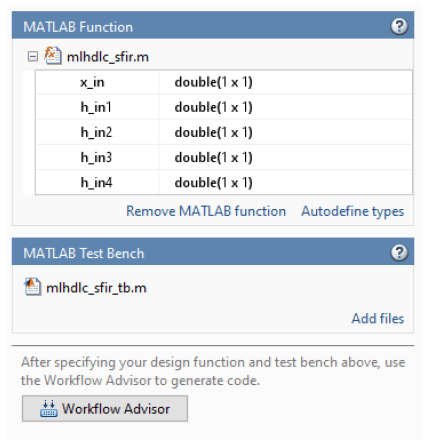# Get Started with MATLAB to HDL Workflow

This example shows how to create an HDL Coder™ project and generate code from your MATLAB® design. In this example, you:

1. Create a MATLAB HDL Coder project.

2. Add the design and test bench files to the project.

3. Start the HDL Workflow Advisor for the MATLAB design.

4. Run fixed-point conversion and HDL code generation.

### FIR Filter MATLAB Design

The MATLAB design `mlhdlc_sfir` is a simple symmetric FIR filter.

```design_name = 'mlhdlc_sfir'; testbench_name = 'mlhdlc_sfir_tb'; ```

Review the MATLAB design.

```open(design_name); ```
```%%%%%%%%%%%%%%%%%%%%%%%%%%%%%%%%%%%%%%%%%%%%%%%%%%%%%%%%%%%%%%%%%%%%%%%%%% % MATLAB design: Symmetric FIR Filter % % Introduction: % % We can reduce the complexity of the FIR filter by leveraging its symmetry. % Symmetry for an n-tap filter implies, coefficient h0 = coefficient hn-1, % coefficient, h1 = coefficient hn-2, etc. In this case, the number of % multipliers can be approximately halved. The key is to add the % two data values that need to be multiplied with the same coefficient % prior to performing the multiplication. % % Key Design pattern covered in this example: % (1) Filter states represented using the persistent variables % (2) Filter coefficients passed in as parameters % Copyright 2011-2019 The MathWorks, Inc. %#codegen function [y_out, delayed_xout] = mlhdlc_sfir(x_in,h_in1,h_in2,h_in3,h_in4) % Symmetric FIR Filter % declare and initialize the delay registers persistent ud1 ud2 ud3 ud4 ud5 ud6 ud7 ud8; if isempty(ud1) ud1 = 0; ud2 = 0; ud3 = 0; ud4 = 0; ud5 = 0; ud6 = 0; ud7 = 0; ud8 = 0; end % access the previous value of states/registers a1 = ud1 + ud8; a2 = ud2 + ud7; a3 = ud3 + ud6; a4 = ud4 + ud5; % multiplier chain m1 = h_in1 * a1; m2 = h_in2 * a2; m3 = h_in3 * a3; m4 = h_in4 * a4; % adder chain a5 = m1 + m2; a6 = m3 + m4; % filtered output y_out = a5 + a6; % delayout input signal delayed_xout = ud8; % update the delay line ud8 = ud7; ud7 = ud6; ud6 = ud5; ud5 = ud4; ud4 = ud3; ud3 = ud2; ud2 = ud1; ud1 = x_in; end ```

### FIR Filter MATLAB Test Bench

A MATLAB testbench `mlhdlc_sfir_tb` exercises the filter design.

```open(testbench_name); ```
```%%%%%%%%%%%%%%%%%%%%%%%%%%%%%%%%%%%%%%%%%%%%%%%%%%%%%%%%%%%%%%%%%%%%%%%%%% % MATLAB test bench for the FIR filter %%%%%%%%%%%%%%%%%%%%%%%%%%%%%%%%%%%%%%%%%%%%%%%%%%%%%%%%%%%%%%%%%%%%%%%%%% % Copyright 2011-2019 The MathWorks, Inc. clear mlhdlc_sfir; T = 2; dt = 0.001; N = T/dt+1; sample_time = 0:dt:T; df = 1/dt; sample_freq = linspace(-1/2,1/2,N).*df; % input signal with noise x_in = cos(2.*pi.*(sample_time).*(1+(sample_time).*75)).'; % filter coefficients h1 = -0.1339; h2 = -0.0838; h3 = 0.2026; h4 = 0.4064; len = length(x_in); y_out = zeros(1,len); x_out = zeros(1,len); for ii=1:len data = x_in(ii); % call to the design 'mlhdlc_sfir' that is targeted for hardware [y_out(ii), x_out(ii)] = mlhdlc_sfir(data, h1, h2, h3, h4); end figure('Name', [mfilename, '_plot']); subplot(3,1,1); plot(1:len,x_in,'-b'); xlabel('Time (ms)') ylabel('Amplitude') title('Input Signal (with noise)') subplot(3,1,2); plot(1:len,y_out,'-b'); xlabel('Time (ms)') ylabel('Amplitude') title('Output Signal (filtered)') freq_fft = @(x) abs(fftshift(fft(x))); subplot(3,1,3); semilogy(sample_freq,freq_fft(x_in),'-b'); hold on semilogy(sample_freq,freq_fft(y_out),'-r') hold off xlabel('Frequency (Hz)') ylabel('Amplitude (dB)') title('Input and Output Signals (Frequency domain)') legend({'FilterIn', 'FilterOut'}, 'Location','South') axis([-500 500 1 100]) ```

### Test the MATLAB Algorithm

To avoid run-time errors, simulate the design by using the test bench.

```mlhdlc_sfir_tb ```### Create an HDL Coder Project

To create an HDL Coder project:

1. In the MATLAB Editor, on the Apps tab, select HDL Coder. Enter `sfir_project` as Name of the project.

To create a project at the MATLAB command prompt, run this command:

```coder -hdlcoder -new sfir_project ```

A `sfir_project.prj` file is created in the current folder.

2. For MATLAB Function, click the Add MATLAB function link and select the FIR filter MATLAB design `mlhdlc_sfir`. Under the MATLAB Test Bench section, click Add files and add the MATLAB test bench `mlhdlc_sfir_tb.m`.

3. Click Autodefine types and use the recommended types for the MATLAB design. The code generator infers the input types from the MATLAB test bench.### Run Fixed-Point Conversion and HDL Code Generation

1. Click the Workflow Advisor button to start the HDL Workflow Advisor.

2. Right-click the HDL Code Generation task and select Run to selected task.

The code generator runs the Workflow Advisor tasks to generate HDL code for the filter design.

• Translate your floating-point MATLAB design to a fixed-point design. To examine the generated fixed-point code from the floating-point design, click the Fixed-Point Conversion task. The generated fixed-point MATLAB code opens in the MATLAB editor. For details, see Floating-Point to Fixed-Point Conversion.

• Generate HDL code from the fixed-point MATLAB design. By default, HDL Coder generates VHDL® code. To examine the generated HDL code, click the HDL Code Generation task, and then click the hyperlink to `mlhdlc_sfir_fixpt.vhd` in the Code Generation Log window. To generate Verilog® code, in the HDL Code Generation task, select the Advanced tab, and set Language to `Verilog`. For more information and to learn how to specify code generation options, see MATLAB to HDL Code and Synthesis.